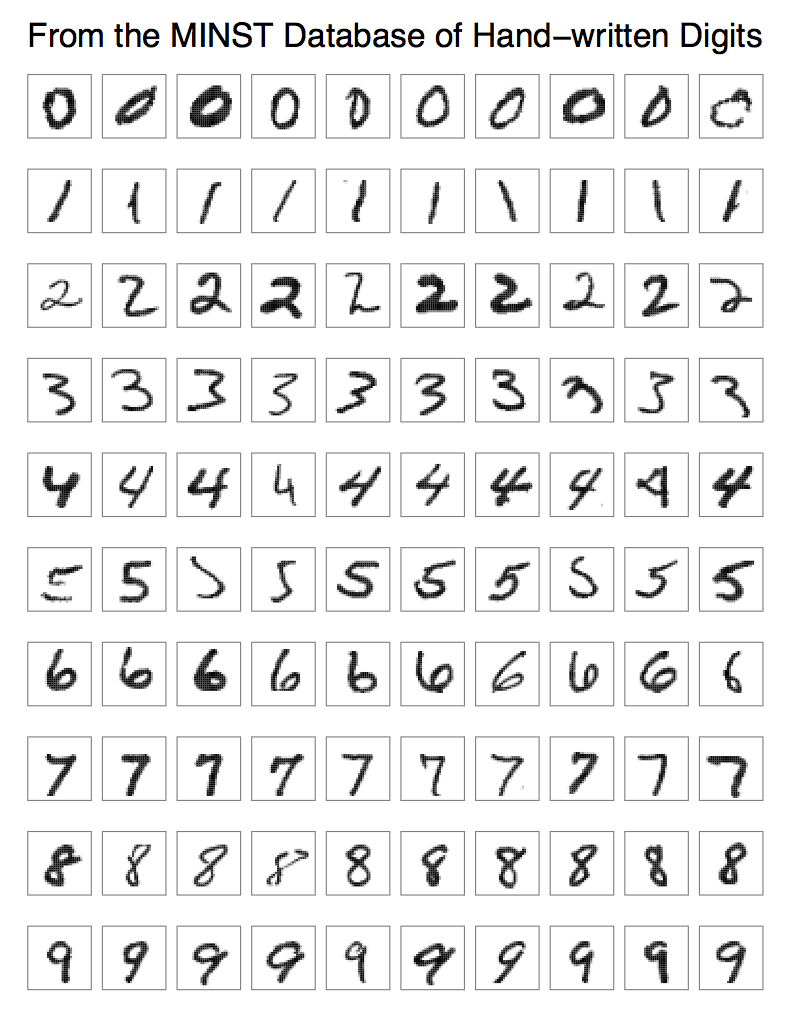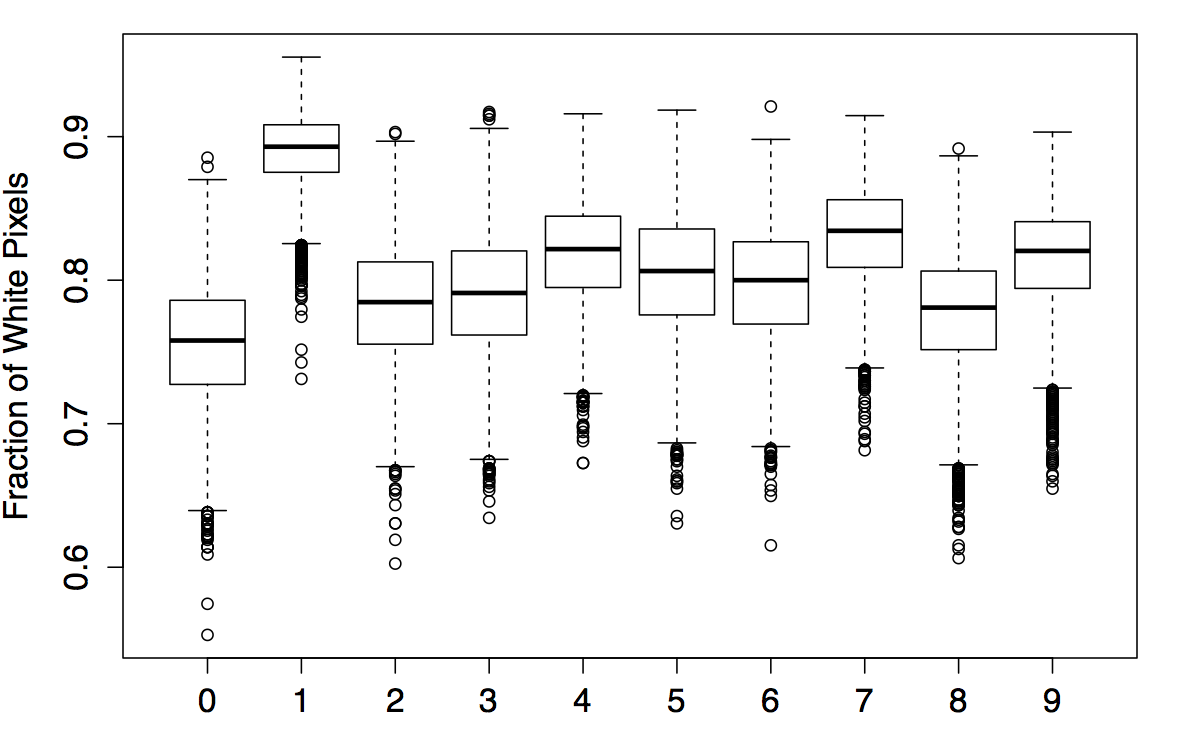In Classification of Hand-written Digits (1), I qualitatively described the machine learning task of classification and sketched out two classic examples, then went into more detail about another well-known example: the classification of hand-written digits. The challenge here is to program a classifier that correctly predicts the value represented in a scanned image of a hand-written digit.

### Analysis Strategy

In any analysis, it pays to have a sensible strategy for how to proceed. I typically go about a machine learning analysis like this:

• Plot and summarize training data to facilitate understanding of the problem you’re trying to solve; ideally, this can reveal underlying relationships in the data and point you toward avenues for further analysis. It’s generally useful to plot full distributions for each feature variable (plus interesting multivariate combinations) as well as calculate the usual summary statistics (mean, variance, skewness, etc.).
• Choose a learning algorithm and implement a basic version that fits your problem/dataset and produces a baseline result to which you can compare subsequent iterations. The choice of algorithm is important but subjective, since for a given task there may be many valid options. Implementing a bare-bones version first is relatively quick and easy, and gives you an idea of how well the algorithm works with your problem/dataset. If it doesn’t work out, for whatever reason, at least you haven’t wasted your time implementing all the bells and whistles!
• Optimize algorithm parameters to improve upon your baseline performance. Obviously the tweakable parameters depend on the algorithm, e.g. the number of hidden layers in an artificial neural network, the number of neighbors to include in a nearest-neighbor algorithm, etc. A common technique for estimating your model’s prediction error is cross-validation, in which only a fraction of the training set is used to train your model, and the remainder (called the validation set) is used to verify your model’s predictions using the actual values. The idea is to choose model parameters that minimize prediction errors.
• Run optimal model on test data to get your final result, and interpret/predict away! If you’ve done a good job in steps 1–3, you should feel confident that the result is on solid statistical footing.

The specifics of one’s analysis strategy depend on the problem at hand, of course, but in general, what I’ve written above will get you moving in the right direction. :)

### Visualizing Hand-written Digits

As it turns out, there’s not a whole lot to visualize in the hand-written digits training set. Each digit (class) is represented by a few thousand examples, and one of the biggest challenges is in correctly accounting for the variability within each class. So, on that note, I produced a plot showing ten examples of each of the ten digits:Made in R with the ggplot package, using facet_wrap and geom_tile.

Besides the obvious variations in shape (e.g. 4s closed/open, 7s with/without hanging lines or cross-bars, etc.), one thing I noticed is that some examples are much bolder than others. To see if that varied systematically by digit (which could potentially be a useful discriminating feature!), I plotted the mean pixel brightness (on a scale from 0 to 255, i.e. white to black) for each class:Boxplot made using R's base graphics package, showing min/max values, lower/upper quartiles, and medians.

In retrospect, this wasn’t particularly insightful, since most digits (with the exception of 1 and maybe 7) are in the same low range of values, owing to the white background on which the black digits are drawn. It did, however, point me to a potentially more interesting variable: the fraction of white pixels in each 28x28 pixel image, which I show here:Another basic boxplot made in R. Btw, those individual points are outliers.

You can see that 1s are about 90% white (empty) pixels, and the other digits are typically more than 75% empty pixels. Why is this interesting? Well, each pixel is treated as a discriminating feature, so the full feature-space of the dataset is 28x28 = 784-dimensional. Seems like an awful lot for telling apart ten classes of digits, right? If a pixel is almost always white for all digits — e.g. corner pixels — it won’t be useful as a feature. Actually, the same can be said for pixels that are almost always black. To generalize this, then, we can say that features with near-zero variance (a measure of the spread of a sample of numbers) across all classes will probably not be useful in discriminating between those classes. Let’s just tuck that bit of insight into our back pockets for now…

Lastly, I wanted to see how digits varied in height and width. One way is to look at the maximum horizontal and vertical ranges spanned by the digits — that is, the first and last columns and rows in [1, 28] with at least one non-white pixel. Here’s a plot of the maximum width for each class of digit:

As you might expect, 1s and 9s tend to be the narrowest, while 0s, 2s, and 5s tend to be the widest. There’s a hard upper bound at 19 pixels because each digit in the MINST database was first normalized to 20x20 pixels in size then centered in a larger 28x28 pixel image. Would this value be useful as an additional discriminator? Eh, probably not, but it was worth a shot. Even less useful was the distribution of heights, since the aforementioned normalization of the digits meant that nearly all digits are exactly 19 pixels tall. I won’t waste your time with the plot.

At this point I could have continued visualizing various aspects of the training data, such as the number of end points or line crossings of each digit or the correlation between individual pixels, but I didn’t feel that it would be particularly useful. So, in the next post, I move on to Step 2 of my analysis strategy.